## Integration Techniques

Important Formulae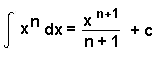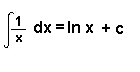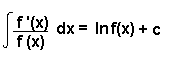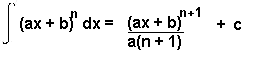Rewriting the Integrand

Dividing

If you are asked to integrate a fraction, try multiplying or dividing the top and bottom of the fraction by a number.

Example

####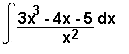If we divide everything on the numerator and everything on the denominator by x2, we get:

= ∫ (3x – 4x-1 – 5x-2) dx
= 3x2 – 4lnx + 5x-1 + c
2

Split into Partial Fractions

Sometimes it will help if you split a fraction up before attempting to integrate. This can be done using the method of partial fractions.

Example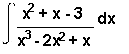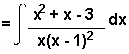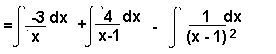(Here we split the fraction into partial fractions)

= -3lnx + 4ln(x - 1) + (x - 1)-1 + c

Using Trigonometric Formulae

When integrating trigonometric expressions, it will often help to rewrite the integral using trigonometric formulae.

Example

∫ cos2x dx

cos2x = 2cos2x - 1
cos2x = ½ (cos2x + 1)

∫ cos2x dx = ½ ∫ (cos2x + 1) dx
= ½ ( ½ sin2x + x) + c
= ¼ sin2x + ½ x + c